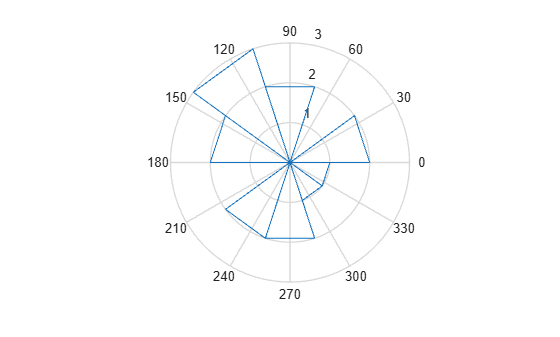# rose

Angle histogram plot

`rose` is not recommended. Use `polarhistogram` instead.

## Syntax

```rose(theta) rose(theta,x) rose(theta,nbins) rose(ax,...) h = rose(...) [tout,rout] = rose(...) ```

## Description

`rose(theta)` creates an angle histogram, which is a polar plot showing the distribution of values grouped according to their numeric range, showing the distribution of `theta` in 20 angle bins or less. The vector `theta`, expressed in radians, determines the angle of each bin from the origin. The length of each bin reflects the number of elements in `theta` that fall within a group, which ranges from 0 to the greatest number of elements deposited in any one bin.

`rose(theta,x)` uses the vector `x` to specify the number and the locations of bins. `length(x)` is the number of bins and the values of `x` specify the center angle of each bin. For example, if `x` is a five-element vector, `rose` distributes the elements of `theta` in five bins centered at the specified `x` values.

`rose(theta,nbins)` plots `nbins` equally spaced bins in the range `[0,2*pi]`. The default is `20`.

`rose(ax,...)` plots into the axes `ax` instead of the current axes (`gca`).

`h = rose(...)` returns the handle of the line object used to create the graph.

`[tout,rout] = rose(...)` returns the vectors `tout` and `rout` so `polar(tout,rout)` generates the histogram for the data. This syntax does not generate a plot.

## Examples

collapse all

Create an angle histogram of values between 0 and $2\pi$. Distribute the data among 10 bins.

```theta = [0.4 1.4 3.1 2.3 0.4 2.5 3.9 2.8 2.3 1.6 4.6 4.5 6.1 3.9 5.1]; rose(theta,10)```## Version History

Introduced before R2006a